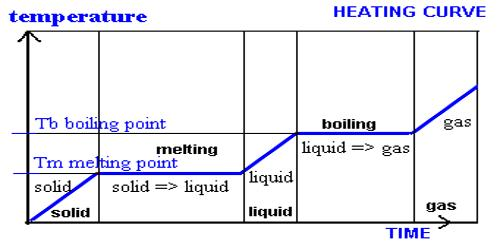Chemistry

# Gas – Solid Systems: Reactions Involving One GasGas – Solid Systems: Reactions Involving One Gas

The following derivation, based on Langmuir’s theory of unimolecular adsorption layer, is made on the assumptions that-

(i) the solid surface has a fixed number of adsorption sites,

(ii) the properties of all adsorption sites are the same,

(iii) each site can hold only one adsorbed molecule,

(iv) the heat of adsorption is independent of the adsorption sites.

Let us consider one cm2 area of a solid surface. Let θ be the fraction covered as a result of adsorption so that the remaining (1 – θ) is bare. Since the number of molecules striking the surface is proportional to the gas pressure P, then Pk1 (1 – θ) gives the rate of adsorption. The number of molecules leaving the surface per unit time, i.e., the rate of desorption is given by k2θ. At equilibrium-

Pk1 (1 – θ) = k2θ … … … (1)

Rearrangement of equation (1) gives,

θ = k1P / (k2 + k1P) … … … (2)

k1 and k2 being two constants. This relation gives rise to two important situations:

(a) Surface nearly bare, i.e., little absorption: Since θ in this case is very small we write k1P (1 – θ) = k1P since (1 – θ) = 1

So that, k1P = k2θ

or, θ = (k1/k2)P

which shows that θ ∞ P. In such cases the reaction rate will be proportional to the first power of the gas pressure and therefore, kinetically first-order, i.e., rate = kP. The decompositions of PH3 on glass. N2O on gold, HI on platinum and AsH3 on glass are some examples of first-order heterogeneous gas reactions.

(b) Surface nearly covered: θ ≈ 1. In such cases,

k1P (1 – θ) = k2

or, (1 – θ) = (k2/ k1) (1/P)

which shows that when adsorption is almost complete the extent of free surface is inversely proportional to pressure P. However, with further adsorption the rate of the reaction becomes independent of pressure, i.e., rate = kP0 = k. This happens because there is no space on the surface where adsorption can take place. Thus the reaction becomes kinetically zero-order. Decomposition of HI on gold surface, and NH3 on tungsten are examples of zero-order reactions.